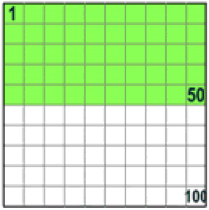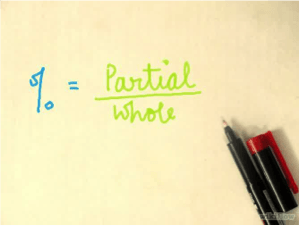Give us feedbackX

# How to Figure Out Percentages

08 Sep 2015Homework Help Online

To know how to figure out percentages, we must first learn about the definition of percentages. This math help will definitely improve your understanding and get you ready to solve your own math problems. You probably have known that percentage uses % symbol, such as 35%, 70%, and 99.9%. Percentage is a way to compare a partial amount of something to its whole amount. In short, you might read % as “out of 100”.Credit: mathisfun

50% (fifty percent) or 50 out of 100. There are 50 green boxes “out of 100” boxes.

25% (twenty five percent) or 25 out of 100. There are 25 green boxes “out of 100” boxes.

## How do we calculate percentages?Credit: wikiHow

In mathematics, percentage means partial divided by whole.

Example:

There are 10 apples in the basket, but 3 of them are rotten. What’s the percentage of fresh apples are in the basket?

Solution:

The partial amount → fresh apples: 10 – 3 = 7 apples.

The whole amount total apples in the basket: 10 apples.

% = 7/10 = 0.7

So, 0.7% of the total apples are fresh.

## How to calculate other variations of percentage problems?

Often, there are other variations of problems which require you to use these formulas:Credit: wikiHow

Example:

1. 5% of 100 students is …
Solution:
100 is the whole amount. We are asked to find the partial amount
5/100 x 100 = 5 students
or you can change 5% into decimal first. 5% = 0.05
0.05 % 100 = 5 students
2. If 35% of the total students in the class are 70, how many students are in the class?
Solution:
70 is the partial amount. We are asked to find the whole amount.
70/35% = 70/35 x 100 = 200 students
or you can change 35% into decimal first. 35% = 0.35
70/0.35 = 200 students

In real life, we’ll often see the application of percentages starting from your allowance increase, interest rate, and discount! To calculate a discount, you have to know first how to figure out percentages.Credit: wikiHow

If the shirt price is \$50, then how much should you pay for the shirt? The discount is 30% x \$50 = 30/100 x \$50 = 3/10 x \$50 = \$15. Therefore, you need to pay \$35. Or you can just calculate 70% x \$50 = \$15. It’s as simple as that when you have mastered how to figure out percentages!

## Other recommended websites to read:

https://www.mathsisfun.com/percentage.html

As a starter, it’s good to read this site as it defines percentages clearly along with some examples. To check your understanding, you may do the exercises.

http://www.wikihow.com/Work-With-Percentages

For variations of percentage problems, go to this webpage. There’s even a video you can watch for learning.

http://math.com/school/subject1/lessons/S1U1L7GL.html

You will find the definition about percentages, examples, and variations of problems. Start from basic, in-depth learning, and then workouts.

http://www.wikihow.com/Calculate-Percentages

This web will provide you with real life illustrations of how to calculate percentage. There are several examples and workouts with solutions to help you, too.

This page contains several brief illustrations of percentages and fractions, including how to convert them. You can use it to understand better of how the comparison works.

Related Posts

Testimonials

• Jason L.

I have a lot of questions that need to be answered. While searching online, I found the site of StudyFAQ and it is all worth it. The tutor is very kind and I’ve got everything I needed. Thank you so much.

• Garry T.

Tutor did a great job. The process was very fast and efficient. I didn’t know that there is a quality and reliable tutoring service provider online. I can now start with my assignment.

• Margret O.

I was really having a hard time finding an expert who can help me with my topic. Thanks to StudyFAQ.com, I can start working on my assignment now.